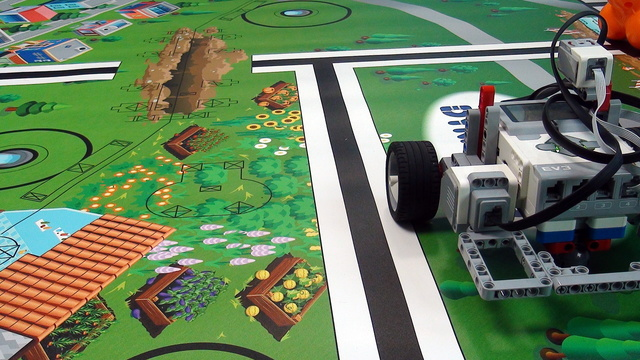В момента ресурсът е наличен само на английски

# Data logging the time between taking samples of the EV3 Gyro sensor ProPreview

After we take each sample, we perform calculations and these calculations could take different time. It is important to know how much time does it take to perform the calculations. In this video tutorial, we would data log the time and plot the data.

• #663
• 10 Jan 2018
• 4:52

### English

In this video we'll discuss a very important aspect from the program for keeping straight that includes the integral part and this is the time between the samples because we know that when you are experimenting with this program you must understand the different parts of the program and an important part is the time between the different samples. So, let's discuss this before we even start implementing the final program. Right here in the program we are recording the values of the gyro sensor and this happens in a loop. So, we record 1 value, a second value, a third value, a forth value and in the meantime after the recording of the value we are doing some calculations and there are some blocks that are executed and something happens between the recordings. And how many recordings per second are we doing? To know this we must know how much time this whole loop takes - the time between the different recordings? And we can measure this time. We can actually detect this time and the way we detect it is: We have a timer at the start right here and we just measure the time at the end. And I'll remove this integral recording and we'll open a new file that's called time

and I would like to record the timer in this file.

And we write like this.

And now we'll know how much time this whole calculation takes. And is it a millisecond or half a millisecond or 10 milliseconds and these are important values when you are measuring the power of the robot, when you are measuring the gyro sensor and this is something that I would like to experiment with now. Start the program.

The robot moves forward and now we open the memory of the brick.

And we open this time file > upload - we upload this file to the computer, to the desktop and then we open it again with Libre.

And what we see here are the number of seconds that it takes for the brick to execute all of the instructions between 2 recordings in the file.

So, it takes about 0.005 seconds so these are 5 milliseconds and here we have 9, we have 4, we have 8, we have 9 and we've made about 41 recordings and none of the recordings is more than 10 milliseconds. And this is because yes, we are doing some calculations but the brick is quite fast with these calculations so if you ever get stuck, and you think: Okay, because I'm doing such a calculation the robot is slow or is not responding as it should be, always measure this and you can measure it like this with just a timer and recording into a file and when you measure it, you'll know if the problem is with the calculations or it's something else. Because these calculations could take quite a while if you are doing some loops or complex IFs or etc. But for such straight calculations where you just multiply and divide and sum some values it is quite fast. So, no more than 10 milliseconds is the whole time between this timer right here where we reset the timer, where we take the value of the gyro sensor where we write something to the file, to the time where we do this writing of the file.

## Курсове и занятия включващи този Урок

Този Урок е използван в следните курсове и занятия.• 24
• 102:09
• 3• 5
• 0
• 0
• 3d_rotation 1